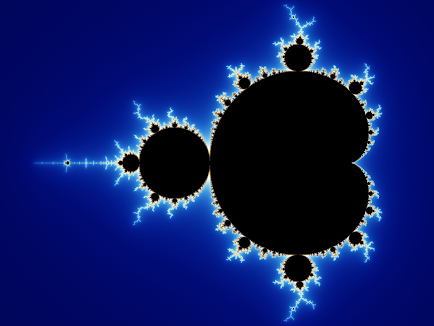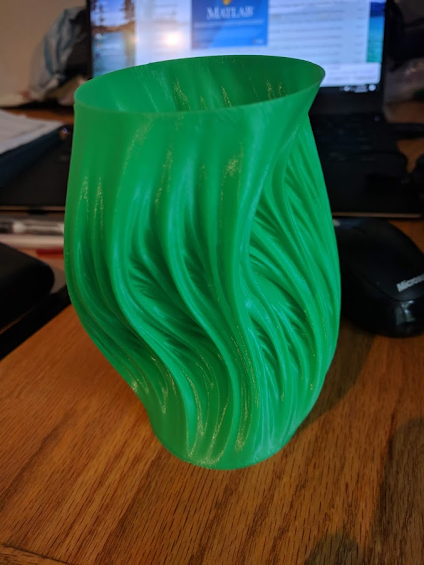### Julia Vase - 3D Prints Generated Using Fractals

A while ago I took a fractals and chaos course. One of the fractals we learned about was the Mandelbrot Set and its respective Julia Sets. The Julia Sets have some interesting properties which make them very easy to 3D print. I made a MATLAB program that generates Julia Set fractals and exports a 3D printable stl file based equations describing a path through the Mandelbrot Set.

The Mandelbrot Set is the set of all complex numbers c that converge when repeatedly plugged into the equation f(c) = z^2 + c starting at z = 0. This deceptively simple equation creates an infinitely complex shape centered roughly around (0,0) in the complex plane.The colors in the Mandelbrot set represent how fast a point diverges

Every point on the Mandelbrot set also has a respective Julia Set. The equation is the same except that c is held constant and the Julia Set is determined by which values of z diverge when c is held constant. The Julia sets generated by groups of points on the Mandelbrot set have some very useful properties:

1. Points near each other will have similar Julia Sets, in other words, the shape of the Julia Set changes "smoothly" if you travel through the Mandelbrot set.

2. Julia sets inside the main bulb of the Mandelbrot set will form one continuous albeit infinitely complex region.Example of the changing Julia Sets as you travel through the Mandelbrot Set

By defining a path that goes through the main bulb of the Mandelbrot Set we can take a snapshot of the Julia Set at fixed intervals along the path. By stacking these snapshots on top of each other we get a 3D shape that has a flat bottom, one main body, and no sharp overhangs. These properties are perfect for 3D printing using vase mode

I was able to approximate the Mandelbrot Set and Julia Sets by checking every point in a grid and plotting it, then removing the internal points. For my 3D print, I used a small elliptical path in the middle of the main bulb of the Mandelbrot Set. The path equation is 0.32*(sin(t*2*pi)) + .3*(cos(t*2*pi)-1)*1i. Code available hereMATLAB script generating the Julia Sets and a point cloud

Using this point cloud I exported an stl file to 3D print. This turned out to be the hardest part of the project; getting MATLAB to properly generate a shape around the point cloud and make a stl file was very difficult. The file also required a good bit of post-processing, I used Meshmixer to smooth out the top and bottom and fix any errors in the stl file.Sliced using vase mode in Cura

Similar designs can be found here